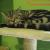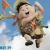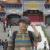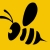## 写给分布式神器fourinone

zhh5919 发布于 2014/05/06 18:31

fourinone号称了集成了hadoop,zk,memcache,mq于一身的四不像超级神器，致力于分布式应用。别的我不懂，今儿聊一下fourinone中的分布式缓存以及分布式文件系统，说到分布式，不得不用到网络通讯，不得不提及RPC，fourinone号称只有100多K，不依赖任何第三方jar，那通讯怎么玩啊！自己用socket或者NIO实现，要知道用NIO实现一个网络通讯框架是很考验技术功底，淘宝的boyan曾经有一篇ppt介绍过NIO的trap和trick.作者当然是绕开这些，使用JDK自带的RMI，RMI这项技术有点老了，我看过JDK的实现还是采用BIO，one request per one thread的方式，序列化采用JDK本身的序列化，性能我不好做评价，因为我没有用过。

好了回归主题，说一说分布式缓存，主流的分布式缓存我们知道用memcached、redis这两个都很出色，fourinone的分布式缓存类似memcached，只支持kv存储。那他是怎么是实现呢？说到kv存储，HashMap是不二之选，对4in1应该也是用的LinkedHashMap,LinkedHashMap,用额外的链表记录访问顺序，LinkedHashMap提供了一个接口removeEldestEntry，使在插入时有机会删除那些最近不活跃的entry，ok继续，分布式缓存，那网络通讯怎么玩的呢？当然是我们前面提到的RMI。至此了解了分布式缓存的实现，简单吧！我相信各位看官看了我的描述自己也能实现了一个自己把玩一下。

继续，说一下这样做的问题，分布式系统几乎是为了高并发而设计的，memcache，redis都是这样。HashMap在高并发下又会怎么样呢？我想你此时脑子里会出现一个场景，这是一个坑，HashMap不是线程安全的，在并发情况下扩容会导致死循环从而导致cpu100%。不了解的可以在coolshell上搜一下，或者问度，这是个致命的问题，我不知道作者是怎么解决的，同步加锁，我没有看到sync，用concurrenthashmap貌似也没用吧。因为concurrenthashmap虽然是线程安全的，但是却没有LRU的功能，如果真要实现,得整合Linkedhashmap和concurrenthashmap的功能于一身，淘宝的人这样做过。再来说一下网络通讯，memcached和redis不约而同的使用非阻塞IO epoll，前者使用libevent，redis在libv的肩膀上简化了轮子。我就不用BIO的RMI了。

说到分布式文件，你会想到那些HDFS，GFS，TFS等等还有好多，还是回到4in1中的fttp，网络通讯怎么玩的呢？fttp，http名字一个字母之差，会不会是http啊，恭喜你猜对了，这时你可能要怀疑，http client好办我用httpurlconnection就可以解决，那http server呢作者内嵌jetty，作者可是号称不依赖任何第三方jar啊难道自己实现一个http server？小伙伴们我们又错了，作者很高端，采用JDK6以后自带的httpserver,关于这个httpserver。这样就可以通过http上传下载文件了，那你说我用jetty和httpclient分分钟就可以分布式文件了，优越感油然而生，信心瞬间爆棚！首先说一下httpserver我读了一下代码，感觉类似还是比较粗放，和Netty没发比，Selector的使用，为了不影响accept事件的阻塞，读写事件都注册到另外一个selector上处理，这样每一个request就会有一个selector，当然作者也才了selector池的处理，使之能够重复使用，但高并发下还是会有很多的selector，而且也会带池的锁开销，再说一下内存管理，每个request都会创建一个buffer，并没有实现内存的管理，这块很粗放，在高并发时GC负担一定很大，感兴趣的小伙伴可以看看netty的实现，作者写过一篇blog说用一个类似伙伴系统和slab内存管理相结合的内存管理方案减少GC。这些且不表，你用过必须制定host以及文件目录的分布式文件系统吗，读写文件时，必须清楚地知道文件在那台机器的那个目录下，orz，这样就要崩溃了

聊了这么多，小伙伴们也可能觉得我对4in1有偏见，其实不然，我只是觉得作者把这些类似玩具的东东开源并推广给大家，并号称分布式，有时也冠以淘宝的名号，大家都知道淘宝在国内开源的影响，小伙伴们很容易会从盲从，特别是不了解分布式想向分布式方面发展学习的小伙伴们，请大家要一定要擦亮眼睛啊。国内开源的分布式不多，讲分布式的书也不多，就那么几本，在此向大家推荐杨传辉的那本书《大规模分布式存储》，书中对主流的分布式kv，分布式文件系统，分布式表格数据库等都有详细的介绍。

我曾经加到4in1的qq群，问了一句“4in1在淘宝那些场景下用到了，好的项目是经得起质疑的，实践是检验真理的唯一标准”结果我被踢出了群，在OSC以及ITEYE也看到一些同学问道了相同的问题，结果也是同样的下场，我不禁要问，作者是抱着什么样的态度开源呢，是跟大家分享，给大家带来便利，还是忽悠，然后写书忽悠？

4in1中的并行计算，hadoop没研究过不懂，本文只针对分布式缓存和分布式文件系统，不对之处敬请拍砖

00#### 引用来自“Li_Peng”的评论

000@fourinone 解释了 map是有同步的，Fttp也不是http协议！ 感谢你的指点！ 如上对你的4in1分析有误你给带来的影响道歉。

HaileiZhang这位朋友，之前已经回复过你，你应该去看看心理医生，为什么如此仇恨fourinone。不知道你是年轻无知还是受人唆使，凭借自己的一知半解，到处散播攻击言论，之前你借口的技术问题，是确实不愿回应你的无理取闹。
map如果连最基本的同步都没有，这么多人想挑fourinone毛病早就挑出来了，你应该再回头去学习。
fttp跟http的区别也有专门文章说明，你根据自己的理解认为就是通过http上传下载，至少对http协议要有所了解，通过http附件应该有“multipart/form-data”方式标记，你在源码里有发现吗，如果没有，你应该继续回头去学习。

redis-benchmark -p 9080 -h 10.103.51.57 -t set -d 50 -c 100 -n 1000000 -r 100000000
====== SET ======
1000000 requests completed in 11.48 seconds
100 parallel clients
50 bytes payload
keep alive: 1

97.87% <= 1 milliseconds
99.39% <= 2 milliseconds
99.56% <= 3 milliseconds
99.67% <= 4 milliseconds
99.73% <= 5 milliseconds
99.75% <= 6 milliseconds
99.77% <= 7 milliseconds
99.78% <= 8 milliseconds
99.79% <= 9 milliseconds
99.80% <= 10 milliseconds
99.81% <= 11 milliseconds
99.82% <= 12 milliseconds
99.82% <= 13 milliseconds
99.83% <= 14 milliseconds
99.84% <= 15 milliseconds
99.84% <= 16 milliseconds
99.84% <= 17 milliseconds
99.85% <= 18 milliseconds
99.86% <= 19 milliseconds
99.87% <= 20 milliseconds
99.87% <= 21 milliseconds
99.87% <= 22 milliseconds
99.88% <= 23 milliseconds
99.88% <= 24 milliseconds
99.89% <= 25 milliseconds
99.89% <= 26 milliseconds
99.89% <= 27 milliseconds
99.89% <= 28 milliseconds
99.89% <= 29 milliseconds
99.90% <= 30 milliseconds
99.90% <= 31 milliseconds
99.91% <= 32 milliseconds
99.91% <= 33 milliseconds
99.91% <= 34 milliseconds
99.91% <= 35 milliseconds
99.91% <= 36 milliseconds
99.91% <= 37 milliseconds
99.91% <= 38 milliseconds
99.92% <= 39 milliseconds
99.92% <= 40 milliseconds
99.93% <= 41 milliseconds
99.93% <= 42 milliseconds
99.93% <= 43 milliseconds
99.93% <= 44 milliseconds
99.93% <= 45 milliseconds
99.93% <= 46 milliseconds
99.93% <= 47 milliseconds
99.94% <= 48 milliseconds
99.94% <= 49 milliseconds
99.94% <= 50 milliseconds
99.94% <= 51 milliseconds
99.94% <= 53 milliseconds
99.94% <= 54 milliseconds
99.95% <= 55 milliseconds
99.95% <= 58 milliseconds
99.95% <= 59 milliseconds
99.95% <= 60 milliseconds
99.95% <= 61 milliseconds
99.95% <= 62 milliseconds
99.95% <= 63 milliseconds
99.95% <= 64 milliseconds
99.95% <= 65 milliseconds
99.95% <= 66 milliseconds
99.95% <= 67 milliseconds
99.96% <= 68 milliseconds
99.96% <= 69 milliseconds
99.96% <= 70 milliseconds
99.96% <= 71 milliseconds
99.96% <= 72 milliseconds
99.96% <= 73 milliseconds
99.96% <= 74 milliseconds
99.97% <= 75 milliseconds
99.97% <= 76 milliseconds
99.97% <= 77 milliseconds
99.97% <= 78 milliseconds
99.98% <= 79 milliseconds
99.98% <= 80 milliseconds
99.98% <= 81 milliseconds
99.98% <= 82 milliseconds
99.98% <= 83 milliseconds
99.98% <= 84 milliseconds
99.98% <= 85 milliseconds
99.98% <= 86 milliseconds
99.98% <= 87 milliseconds
99.98% <= 88 milliseconds
99.98% <= 89 milliseconds
99.98% <= 90 milliseconds
99.98% <= 91 milliseconds
99.98% <= 92 milliseconds
99.98% <= 93 milliseconds
99.99% <= 96 milliseconds
99.99% <= 97 milliseconds
99.99% <= 100 milliseconds
99.99% <= 101 milliseconds
99.99% <= 103 milliseconds
99.99% <= 106 milliseconds
99.99% <= 110 milliseconds
99.99% <= 111 milliseconds
99.99% <= 112 milliseconds
99.99% <= 113 milliseconds
99.99% <= 125 milliseconds
99.99% <= 126 milliseconds
100.00% <= 127 milliseconds
100.00% <= 137 milliseconds
100.00% <= 200 milliseconds
100.00% <= 201 milliseconds
100.00% <= 6000 milliseconds
100.00% <= 6000 milliseconds
87092.84 requests per second